### Home > CALC > Chapter 7 > Lesson 7.4.2 > Problem7-185

7-185.
1. No calculator! Find the area represented by each definite integral below. If the integral is improper, rewrite it in "proper" limit notation first. One of these will need to be written as the sum of two improper integrals as the first step. Homework Help ✎

1.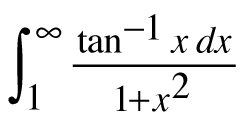2.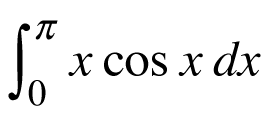3.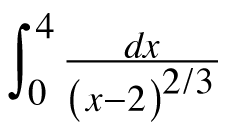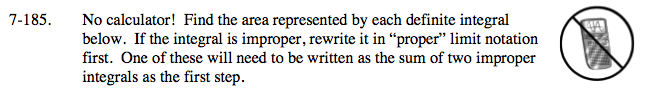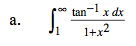Let U = tan−1x.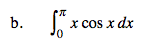Use Integration by Parts;
If f(x) = x and dg = cosx dx,
then df = ___ and g(x) = ____.

Evaluate and solve.

$=f(x)g(x)\Big|_0^\pi-\int_{0}^{\pi }g(x)df$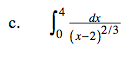Write this integral as the sum of two improper integrals.

$\lim_{a\rightarrow 2^{-}}\int_{0}^{a}\frac{dx}{(x-2)^{\frac{2}{3}}}+\lim_{a\rightarrow 2^{+}}\int_{a}^{4}\frac{dx}{(x-2)^{\frac{2}{3}}}$